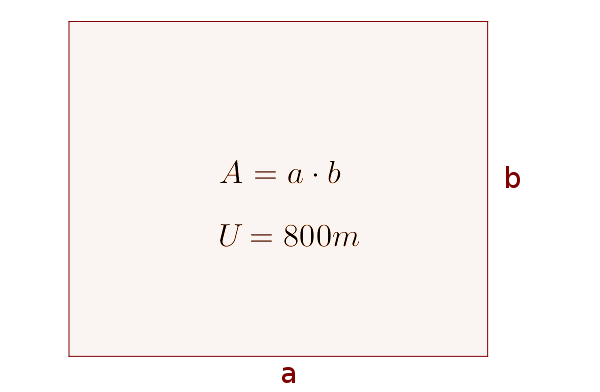Math Application differential calculus Extreme value problem

# Extreme value problem

One type of task that uses differential calculus are optimizations or extreme value problems. An extreme value problem is a kind of optimization problem but only with one constraint. It can be both a maximization problem and a minimization problem.

i

### Hint

Extreme value problems exist when a target size (e.g., surface area, volume, profit, ...) is to become maximum or minimum. This condition is then the main condition.
!

### Remember

In the case of extreme value problems, a function (the objective function) is set up from a main condition and a constraint, whose extreme values are searched for.
i

### Method

1. Main condition
2. Constraint
3. Set up objecitve function
4. Calculate extreme values of the objective function
5. Calculate missing sizes

### Example

The largest possible rectangle area has to be limited with an 800 m fence. Calculate the size of both sides and the area.1. #### Main condition

The area of the rectangle should be maximized. Therefore, this is the main condition and depends on two variables $a$ and $b$.

$A(a, b)=a\cdot b$
2. #### Constraint

There are only 800 m of fence available, which limits the area. This is the perimeter of the rectangle.

$P=2a+2b$
$800=2a+2b$
3. #### Set up objective function

To link both conditions, the constraint is changed to a variable.
$800=2a+2b\quad|-2b$
$800-2b=2a\quad|:2$
$a=\frac{800-2b}2$ $=400-b$

Now this must be used in the main condition and you get the objective function, which is dependent on only one variable.
$A(a, b)=a\cdot b$
$A(b)=(400-b)\cdot b$ $=400b-b^2$
4. #### Calculate extreme values of the objective function

Now you can (as with other functions too) calculate the extreme values of the objective function.
$A(b)=400b-b^2$
$A'(b)=400-2b$

$400-2b=0\quad|-400$
$-2b=-400\quad|:(-2)$
$b=200$

With the second derivation, it is still checked whether the result is actually a maximum point, since the area should be maximum.
$A''(b)=-2$
$A''(200)=-2<0$ => Maximum point
5. #### Calculate missing sizes

$b=200m$

From the (converted) constraint you can now calculate $a$.
$a=400-b$
$a=400-200=200m$

From the main condition (alternatively also with the objective function) the area $A$ can be calculated.
$A(a, b)=a\cdot b$
$A(a, b)=200m\cdot 200m=40.000m^2$